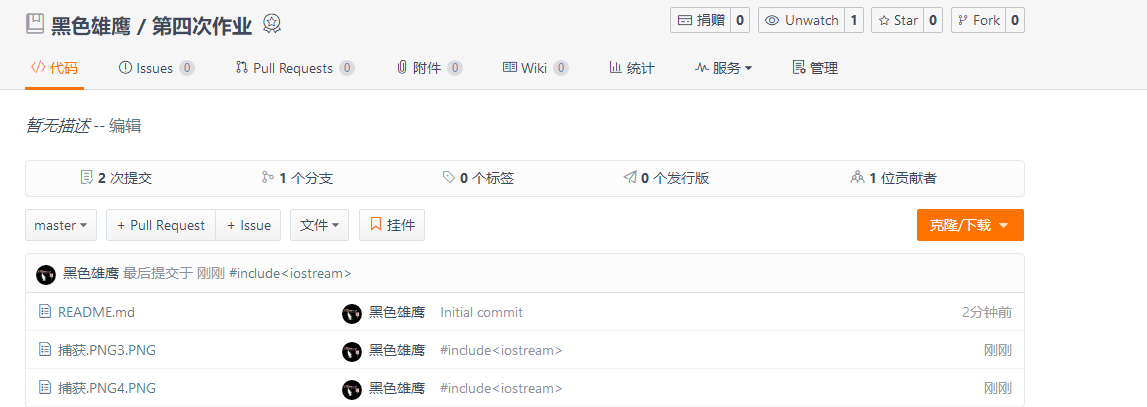## 第04次作业-树

2018-05-05 20:13  黑鹰1096  阅读(472)  评论(0编辑  收藏  举报

# 1.学习总结

## 1.1树结构思维导图# 2.PTA实验作业

• 如果选6-1，只需要描述建树的伪代码。
• 6-4必选

## 2.1 题目1：

### 函数接口定义：

int GetHeight( BinTree BT );


typedef struct TNode *Position;
typedef Position BinTree;
struct TNode{
ElementType Data;
BinTree Left;
BinTree Right;
};


### 裁判测试程序样例：

#include <stdio.h>
#include <stdlib.h>

typedef char ElementType;
typedef struct TNode *Position;
typedef Position BinTree;
struct TNode{
ElementType Data;
BinTree Left;
BinTree Right;
};

BinTree CreatBinTree(); /* 实现细节忽略 */
int GetHeight( BinTree BT );

int main()
{
BinTree BT = CreatBinTree();
printf("%d\n", GetHeight(BT));
return 0;
}
/* 你的代码将被嵌在这里 */


### 输出样例（对于图中给出的树）：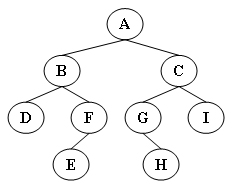4

2.2 设计思路（伪代码或流程图）

f(b)=0

f(b)=Max{f(b->child),f(b->rchild)}+1;

## 2.3 代码截图（注意，截图、截图、截图。代码不要粘贴博客上。不用用···语法去渲染）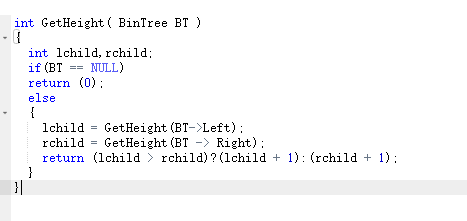## 2.4 PTA提交列表说明。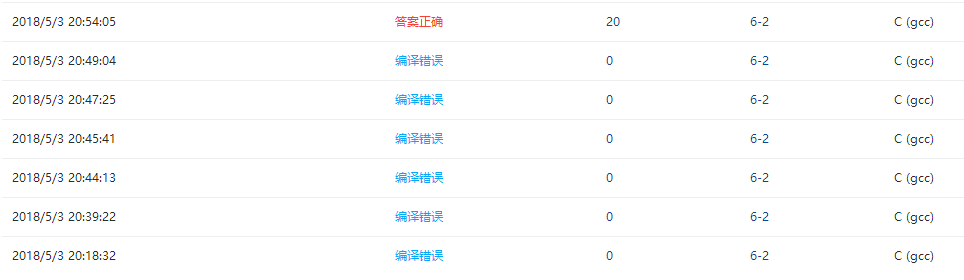## 2.1 题目2：

### 输入样例:

9
ABDFGHIEC
FDHGIBEAC


### 2.2 设计思路（伪代码或流程图）

f（b，x）= NULL

f(b,x) = b

f(b,x) = p

TreeNode*findTree(char*in,char*pre,int length)

## 2.3 代码截图（注意，截图、截图、截图。代码不要粘贴博客上。不用用···语法去渲染）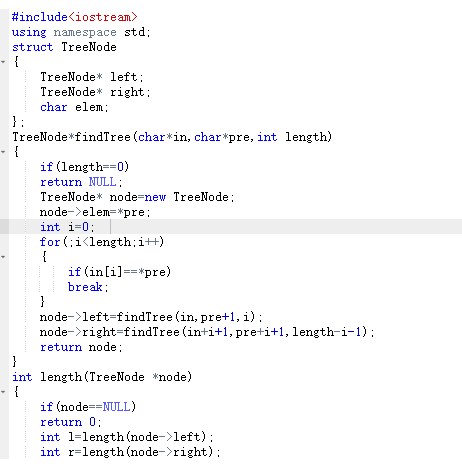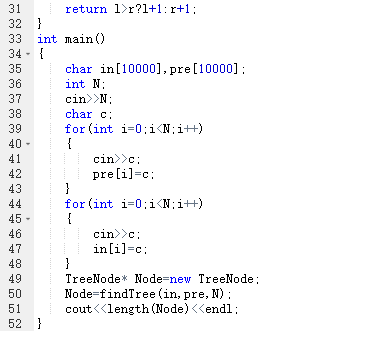## 2.4 PTA提交列表说明。## 2.1 题目3：

#### 6-4 jmu-ds-表达式树（25 分）

• 输入一行中缀表达式，转换一颗二叉表达式树，并求解.
• 表达式只包含+-*/（）运算符，操作数只有一位，且为整数(有兴趣同学可以考虑负数小数，两位数做法)。按照先括号，再乘除，后加减的规则构造二叉树。
• 如图所示是"1+(2+3)*2-4/5"代数表达式对应二叉树，用对应的二叉树计算表达式的值。 转换二叉树如下：### 函数接口定义：

void InitExpTree(BTree &T,string str) ; //建表达式的二叉树
double EvaluateExTree(BTree T);//计算表达式树


T：二叉树 str:输入的表达式

### 裁判测试程序样例：

#include<iostream>
#include<string>
#include<stack>
using namespace std;
typedef struct BiTNode{                             //二叉树的二叉链表存储表示
char data;
struct BiTNode *lchild,*rchild;
}BTNode,*BTree;
void InitExpTree(BTree &T,string str) ; //建二叉表达式树
double EvaluateExTree(BTree T);//计算表达式树
char Precede(char t1,char t2) ;//比较t1,t2运算符优先级函数
int In(char c) ;//判断c是否运算符
void CreateExpTree(BTree &T,BTree a,BTree b,char ch);//建简单二叉树
void DestroyBTree(BTree bt);//销毁树
int main()
{
string str;
BTree T;
getline(cin,str);
InitExpTree(T,str);          //创建表达式树
cout<<EvaluateExTree(T);             //输出值
DestroyBTree(T);
return 0;
}
char Precede(char t1,char t2)
{ /*判断两符号的优先关系 */
char f;
switch(t2)
{
case '+':if(t1=='('||t1=='#')
f='<';
else
f='>';
break;
case '-':if(t1=='('||t1=='#')
f='<';
else
f='>';
break;
case '*':if(t1=='*'||t1=='/'||t1==')')
f='>';
else
f='<';
break;
case '/':if(t1=='*'||t1=='/'||t1==')')
f='>';
else
f='<';
break;
case '(':if(t1!=')')
f='<';
break;
case')':if(t1=='(')
f='=';
else
f='>';
break;
case'#':if(t1=='#')
f='=';
else
f='>';
}
return f;
}
int In(char c)
{ /* 判断c是否为运算符 */
switch(c)
{
case '+':
case'-':
case'*':
case '/':
case'#':
case '(':
case')':return 1;break;
default:return 0;
}
}
void CreateExpTree(BTree &T,BTree a,BTree b,char ch){           //简单二叉树的创建
T=new BTNode;
T->data=ch;
T->lchild=a;
T->rchild=b;
}
void DestroyBTree(BTree bt)//销毁树
{
if(bt!=NULL)
{
DestroyBTree(bt->lchild);
DestroyBTree(bt->rchild);
delete bt;
}
}
/* 请在这里填写答案 */


### 输入样例：

1+2*3


## 2.3 代码截图（注意，截图、截图、截图。代码不要粘贴博客上。不用用···语法去渲染）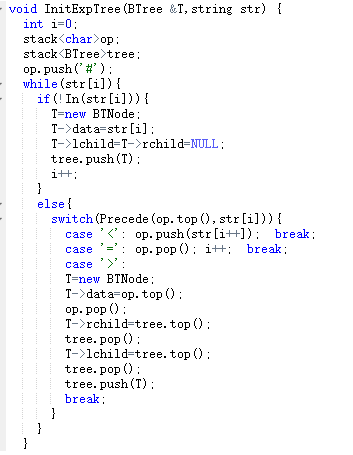## 2.4 PTA提交列表说明。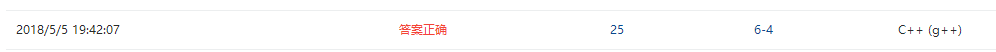# 3.1 PTA排名截图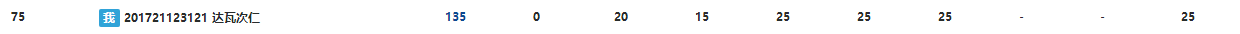## 3.2 我的总分：

1.5分

# 5. 代码Git提交记录截图